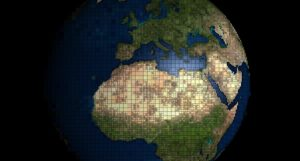## Putting It Together: Historical Counting SystemsComputers speak binary.  The binary, or base 2, positional number system uses only two digits, 0 and 1, which makes it ideal for computers whose basic components typically exist in two states, off (0) or on (1).  However, binary numbers can be very tedious for human readers to interpret.  For example, can you figure out what decimal number is represented by the following binary number?

$110111101100000101011001000111111$

There are 33 digits in this numeral!  We may estimate roughly how big this number is by working out just the first few digits (we’ll work out just four digits, but you could go further if you wanted to).

$1 \times 2^{32} + 1 \times 2^{31} + 0 \times 2^{30} + 1 \times 2^{29}$

$= 4,294,967,296 + 2,147,483,648 + 0 + 536,870,912$

$\approx 7,000,000,000$

This number, about 7 billion, represents the world population on January 1st, 2017 according to the website, Population.City.

Now if we wanted to work out the decimal value of this number down to the last digit, there is a nice shortcut.  First let’s separate the digits into groups of four.

$1\; 1011\; 1101\;1000\; 0010\; 1011\; 0010\; 0011\; 1111$

Each group may now be thought of as a numeral in base $2^4 =16$.  The base 16, or hexadecimal, number system requires 16 digits.  In particular, the digits corresponding to decimal 10 through 15 are usually written as the letters A through F, as indicated in Table I.

Table I:  Hexadecimal digits and their equivalent decimal representations.

 Hexa- decimal 0 1 2 3 4 5 6 7 8 9 A B C D E F Decimal 0 1 2 3 4 5 6 7 8 9 10 11 12 13 14 15

Furthermore, there is a one-to-one correspondence between every possible 4-digit binary expression and the hexadecimal digits, as shown in Table II.  It is this correspondence that makes hexadecimal a natural medium for writing large binary numbers in a more human-readable way.

 Binary Hex. Binary Hex. Binary Hex. Binary Hex. 0000 0 0100 4 1000 8 1100 C 0001 1 0101 5 1001 9 1101 D 0010 2 0110 6 1010 A 1110 E 0011 3 0111 7 1011 B 1111 F

So let’s see what the population of the Earth looks like in hexadecimal notation.  Note that the leading “1” in our numeral should be interpreted as “0001.”

 0001 1011 1101 1000 0010 1011 0010 0011 1111 1 B D 8 2 B 2 3 F

The resulting hexadecimal number, 1BD82B23F, is much easier to write down and to work with than the original binary representation, at least for us non-computers.

Finally, let’s figure out the exact decimal representation of the number using what we have learned in this module about place-value systems.

 Digit Place value Computed values 1 $16^8$ $1 \times 16^8 = 4,294,967,296$ B $16^7$ $11 \times 16^7 = 2,952,790,016$ D $16^6$ $13 \times 16^6 = 218,103,808$ 8 $16^5$ $8 \times 16^5 = 8,388,608$ 2 $16^4$ $2 \times 16^4 = 131,072$ B $16^3$ $11 \times 16^3 = 45,056$ 2 $16^2$ $2 \times 16^2 = 512$ 3 $16$ $3 \times 16 = 48$ F $1$ $15 \times 1 = 15$ Total: $7,474,426,431$

Almost seven and a half billion people call this planet home.  That’s a lot of people regardless whether the amount is expressed in decimal, hexadecimal, or binary notation.